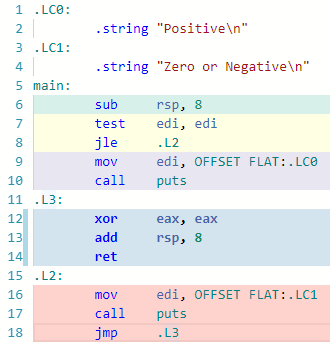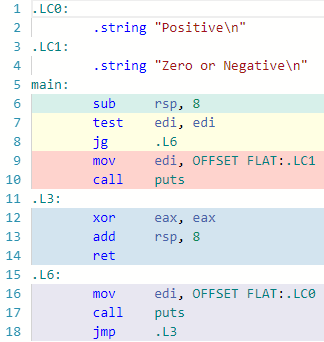# likely — unlikely directives

## Assisting the compiler in optimizing if conditions

A look at the Linux kernel code will show many if conditions enclosed in likely and unlikely macros. These macros invoke compiler directives that give the compiler a hint on the code leg that should be optimized for performance.

For example, the following translation lookaside buffer (TLB) insert function from the Linux kernel code contains an if-condition with the `likely` directive. Here the author is telling the compiler that it is very likely that the TLB entry lookup will fail.

`static void tlb_entry_insert(unsigned int pd0, pte_t pd1){ unsigned int idx; idx = tlb_entry_lkup(pd0); if (likely(idx & TLB_LKUP_ERR))  write_aux_reg(ARC_REG_TLBCOMMAND, TLBGetIndex); write_aux_reg(ARC_REG_TLBPD1, pd1); write_aux_reg(ARC_REG_TLBCOMMAND, TLBWrite);}`

Similarly, developers can use the `unlikely` macro to signal to the compiler to optimize the code for the else-leg being taken. Refer to the following example from the Linux kernel code on how the `unlikely` macro is used. Here the compiler is being instructed that it is unlikely that 32 or more pages are getting flushed at the same time. The compiler should optimize for less than 32 pages.

`void local_flush_tlb_kernel_range(unsigned long start,                                   unsigned long end){ unsigned long flags;if (unlikely((end - start) >= PAGE_SIZE * 32)) {  local_flush_tlb_all();  return; }start &= PAGE_MASK;local_irq_save(flags);while (start < end) {  tlb_entry_erase(start);  start += PAGE_SIZE; }utlb_invalidate();local_irq_restore(flags);}`

# How does the compiler optimize for the likely and unlikely directives?

## Examine the code generation for if and else

Let’s use a simple example to understand the optimization. The following code contains a single if-else. The corresponding assembly is shown on the right side. Click on the image to open the code in the Compiler Explorer.Default code generated without the use of the likely and unlikely directives

Let’s trace the path in the assembly code for the if and else branches:

Code execution when argc > 0 (if-leg is taken)

1. `sub rsp,8` — Allocate space on the stack.
2. `test edi, edi` — Test the value of `argc`.
3. `jle .L2` — Jump if less than equal to. This jump is not taken.
4. `mov edi, OFFSET FLAT:.LC0` — Copy the pointer to the string `"Positive\n"` into `edi` register.
5. `call puts` — Call the puts function.
6. `xor eax, eax` — Set `eax`to 0 (compilers use a self XOR as it is faster than the instruction for setting `eax` to 0).
7. `add rsp, 8` — Release space on the stack.
8. `ret` — return from `main`.

Code execution when argc ≤ 0 (else-leg is taken)

1. `sub rsp,8` — Allocate space on the stack.
2. `test edi, edi` — Test the value of `argc`.
3. `jle .L2` — Jump if less than equal to. This jump is taken.
4. `.L2` Jump is taken to label `.L2`.
5. `mov edi, OFFSET FLAT:.LC1` — Copy the pointer to the string `"Zero or Negative\n"` into `edi` register.
6. `call puts` — Call the puts function.
7. `.L3`Unconditional jump to `.L3`.
8. `xor eax, eax` — Set `eax`to 0.
9. `add rsp, 8` — Release space on the stack.
10. `ret` — return from `main`.

From the code trace, it is clear that the compiler has optimized the if leg to execute the code without any branching. The else leg encounters two extra branches (step 4 and 7 in the else leg trace — argc ≤ 0).

## Optimize the else leg using the unlikely directive

What if the application requires the else leg to be optimized? This is achieved by introducing the `unlikely` macro in the if-condition. Click on the image to open the code in the Compiler Explorer.Code generated when the unlikely directive is used

We can see now that compiler has flipped the optimization. Now the else leg executes without a branch being taken. The if leg encounters two branches.

Code execution when argc ≤0 (else-leg is taken)

1. `sub rsp,8` — Allocate space on the stack.
2. `test edi, edi` — Test the value of `argc`.
3. `jg .L6` — Jump if greater. This jump is not taken.
4. `mov edi, OFFSET FLAT:.LC1` — Copy the pointer to the string `"Zero or Negative\n"` into `edi` register.
5. `call puts` — Call the puts function.
6. `xor eax, eax` — Set `eax`to 0.
7. `add rsp, 8` — Release space on the stack.
8. `ret` — return from `main`.

Code execution when argc >0 (if-leg is taken)

1. `sub rsp,8` — Allocate space on the stack.
2. `test edi, edi` — Test the value of `argc`.
3. `jg .L6` — Jump if greater. This jump is taken.
4. `.L6` Jump is taken to label `.L6`.
5. `mov edi, OFFSET FLAT:.LC0` — Copy the pointer to the string `"Positive\n"` into the`edi` register.
6. `call puts` — Call the puts function.
7. `.L3`Unconditional jump to `.L3`.
8. `xor eax, eax` — Set the`eax`to 0.
9. `add rsp, 8` — Release space on the stack.
10. `ret` — return from `main`.

## Using the likely directive optimizes the if-leg of the code

In this example, using the `likely` macro generates the same code as not specifying any directive. The if-leg is optimized to execute without any branching.

## Definition of the likely and unlikely macros

Currently, `likely` and `unlikely` macros need to be defined to invoke the `__builtin_expect` function.

`#define likely(x)       __builtin_expect(!!(x), 1)#define unlikely(x)     __builtin_expect(!!(x), 0)`

# likely and unlikely attributes in C++ 20

If the use of the `likely` and `unlikely` macros seemed a bit ad hoc, watch out for the C++ 20 `[[likely]]` and `[[unlikely]]` attributes. These attributes would also be supported in switch-statement.

`int foo(int i) {    switch(i) {               case 1: handle1();                       break;    [[likely]] case 2: handle2();                       break;    }}`

The C++ 20 proposal for the `[[likely]]` and `[[unlikely]]` describes the advantages of giving direct hints to the compiler.

# Performance impact of the likely and unlikely hints

What is the performance gain from using these macros? Let’s use an example from the C++ 20 proposal to understand the performance difference caused by an update to the `clamp` function in the example.

Let’s examine the code of the function with and without the `unlikely` hint.

## Without hint

Here the compiler generates pretty tight code with very little room for optimization.

1. `xor eax, eax` — Default return value to 0
2. `test edi, edi` — Check if `i` is 0
3. `cmp edi, 65535` — Compare `i` with 65535
4. `mov eax, -1` — Default return value to 65535
5. `cmovle eax, edi` — Copy `i` into the return value if `i` ≤ 65535
6. `ret` — return

## With hint (out of range is unlikely)

Use of the `unlikely` macro allows the compiler to optimize by removing an instruction from the likely path.

1. `test edi, edi` — Check if `i` is 0
2. `cmp edi, 65535` — Compare `i` with 65535
3. `mov eax, -1` — Default return value to 65535
4. `cmovle eax, edi` — Copy `i` into the return value if `i` is ≤ 65535
5. `ret` — return

From the above comparison, we see that the compiler has removed on instruction from the likely path — `xor eax, eax`.

## Benchmark results

From the benchmark results in the paper, we see that the version of the `clamp` function with the `unlikely` results in a fairly significant reduction in the execution time if the out of range values are between 0.1% and 10%. After that. As expected there is a small penalty to be paid when the data contains values that take the unlikely leg.

# When should I give compiler explicit hints?

In most of your code, you should not sacrifice readability by littering the code with `likely` and `unlikely` macros.

• In most scenarios, the processor’s branch prediction cache does a pretty good job of predicting the likely branch to be taken. This is especially true inside loops.
• That said, the performance-critical innermost loops in the code might see an improvement in performance hints.
• Logging macros might benefit from an `unlikely` macro. This tells the compiler that it is quite unlikely that the logging is enabled. This will help lower the cost of LOG level check if statements that are invisibly included in a lot of your code.
`#define LOG(level, message)  if (unlikely(enabledLogLevel > level))\                                sendLog(message);`

--

--